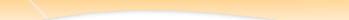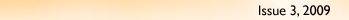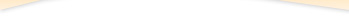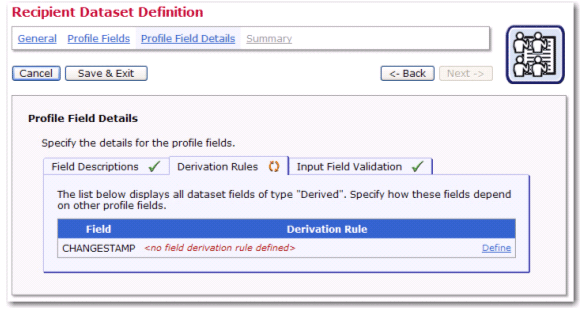Tech Tip: LISTSERV MaestroQ: Is it possible to add a "timestamp" profile field to a dataset that is updated whenever any of the other profile fields are changed? Answer by Johannes Hubert and Holly TaylorLISTSERV Maestro Project Manager and Technical Writer,L-Soft For many data administrators, keeping track of their subscribers' data and its accuracy is a major concern. Simply having a way to keep track of the last date and time that a specific record was updated would be beneficiary. Because of this need, LISTSERV Maestro 4.0-5 and later has made it possible for you to create a "timestamp" that helps you keep your subscriber information up-to-date. This timestamp is created by adding a special derivation rule formula to a derived profile field. To define a timestamp profile field for a specific dataset, go to the Recipient Dataset Details screen for that dataset, and then select the Dataset menu from the Toolbar and select Edit Dataset Settings. The Recipient Dataset Definition wizard opens. On the Profile Fields screen, click the Add Field link. Enter a suitable Name and Display Name, for example "CHANGESTAMP" and "Last Updated". Click the Data Type drop-down arrow and select Derived, and then click the Input Type drop-down arrow and select Hidden. (This input type ensures that subscribers won't see this change timestamp on their pages). Click [Next] to define the derivation rule.On the Profile Field Details screen, click the Derivation Rules tab. For the newly added CHANGESTAMP field, click the Define link.In the Derivation Rule window, set the derivation rule of this new field to the following formula for the derivation rule: If(true, CurrentMillis, Length(FIELD_LIST)) where you replace FIELD_LIST with a "+" concatenation of all other profile fields in your dataset (or hosted list), like this: &FIELD1; + &FIELD2; +&FIELD3; + [etc]... However, you need to observe a few rules depending on each field's type so that this concatenation list results in a correct formula syntax and is accepted by the system: Text and Mandatory field types – Nothing special to observe, just add the field to the list, i.e. write &FIELD; Text and Optional field types – Use the optional-field syntax to add the field, i.e. write [&FIELD; ""] Number and Mandatory field types – Nothing special to observe, just add the field to the list, i.e. write &FIELD; Number and Optional field types – Use the optional-field syntax to add the field, i.e. write [&FIELD; 0] Boolean field types – Wrap the field in the ToNum() function, i.e. write ToNum(&FIELD;) Tracking Permission field types – See "Boolean field types" Single Select (Mandatory or Optional) field types – Nothing special to observe, just add the field to the list, i.e. write &FIELD; Multiple Select (Mandatory or Optional) field types – Wrap the field in the Count() function, i.e. write Count(&FIELD;) Derived field types – Does not need to be mentioned in the formula at all, i.e. just leave it out. For Example Assume that you have the following profile fields: TEXTOPT (optional text)TEXTMAND (mandatory text)NUMOPT (optional number)NUMMAND (mandatory number)BOOL (boolean)SINGLE (single select)MULTI (multiple select) Then, the derivation formula would look like this: If(true, CurrentMillis, Length([&TEXTOPT; ""] + &TEXTMAND; + [&NUMOPT; 0] + &NUMMAND; + ToNum(&BOOL;) + &SINGLE; + Count(&MULTI;))) Or, the same with linebreaks for better readability: ``` If(true, CurrentMillis, Length([&TEXTOPT; ""] + &TEXTMAND; + [&NUMOPT; 0] + &NUMMAND; + ToNum(&BOOL;) + &SINGLE; + Count(&MULTI;) ) ) ``` Restriction: It is not possible to have a derived field in a hosted list that references (in its formula) profile fields that are defined in the dataset. Similarly, it is not possible to have a derived field in a dataset that references (in its formula) profile fields that are defined in any of the hosted lists of the dataset. Therefore, if you have both profile fields in the dataset (i.e. shared fields for all lists) and individual profile fields in a hosted list, then you can not have a change-timestamp for all of these profile fields (from dataset and list) at once. Your only option is to have two change-timestamps: One for the dataset profile fields (defined as a derived field in the dataset, referencing all other dataset profile fields, but no list profile fields), and one for the list profile fields (defined as a derived field in the list, referencing all other list profile fields, but no dataset profile fields). Important Notes about the Derivation Rule Formula It is important to know that a derived field's value is recalculated whenever the value of any of the other profile fields that are mentioned in the derivation rule formula changes. Therefore, what we need to come up with is a formula, whose result is the current time (so that it can be used as a timestamp), but which is also recalculated whenever any of the (other) profile fields are changed. Therefore, the formula must not only result in the current time, but it also must mention all the other profile fields somewhere. This is what the above formula does, using the "If" function: 1. The first argument of the function is the condition. In this case, the condition is set to a constant "true". 2. The second argument of the function is the result that is to be returned if the condition is true. In this case, since the condition is always "true" (see above), the value of the second argument is always the value that is returned by the function. Therefore, we simply set "CurrentMillis" as the second argument, which will have the effect that the second argument is equal to the current time; and, since the second argument will always be returned as the result of the If-function itself (because of the constant "true" condition), the result of the whole If-function (and thus the whole derivation rule formula) will be the current time, just as we need it for our timestamp. 3. The third argument of the function is the result that is to be returned if the condition is false. In this case, since the condition is always "true" (see above), this means that the value of this second argument will never be returned. So we are free to put into this third argument whatever we want (as long as it is syntactically valid and results in a Number). We take advantage of this (i.e. that the third argument is always ignored). As said above, to make the derivation rule recalculate its value (to the current time) each time any of the other profile fields are changed, we need to mention all of these other profile fields somewhere in the formula, but we don't want them to actually influence the return value of the formula (which is supposed to be the current time). And, since the third argument is ignored, we can use it for exactly this purpose, i.e. to mention all the other profile fields without influencing the result of the formula, which is the purpose of the "FIELD_LIST". Note: Notice how we put an additional "Length()" function around the "FIELD_LIST". This ensures that the data type of the value from "FIELD_LIST" is of the Number type to match the data type of "CurrentMillis", which is also Number. This is because the "FIELD_LIST" will be a concatenation of all other profile fields (to "mention" them in the formula); and, the data type of such a concatenation is usually Text, which is why we put the "Length()" function around it to convert the Text type back into a Number type.© L-Soft 2009. All Rights Reserved.